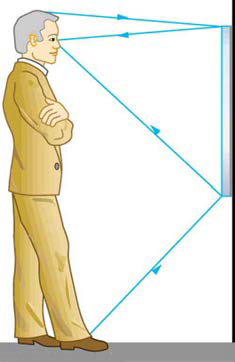×
Get Full Access to College Physics - 1 Edition - Chapter 25 - Problem 1pe
Get Full Access to College Physics - 1 Edition - Chapter 25 - Problem 1pe

×

# Suppose a man stands in front of a mirror as shown inISBN: 9781938168000 42

## Solution for problem 1PE Chapter 25

College Physics | 1st Edition

• Textbook Solutions
• 2901 Step-by-step solutions solved by professors and subject experts
• Get 24/7 help from StudySoup virtual teaching assistantsCollege Physics | 1st Edition

4 5 1 391 Reviews
20
2
Problem 1PE

Suppose a man stands in front of a mirror as shown in Figure 25.50. His eyes are 1.65 m above the floor, and the top of his head is 0.13 m higher. Find the height above the floor of the top and bottom of the smallest mirror in which he can see both the top of his head and his feet. How is this distance related to the man’s height?Figure 25.50 A full-length mirror is one in which you can see all of yourself. It need not be as big as you, and its size is independent of your distance from it.

Step-by-Step Solution:
Step 1 of 3

Solution 1PE

By the law of reflection and ray tracing, the angle of incidence is equal to the angle of reflection, so the top of the mirror must extend to at least halfway between his eyes and the top of his head and the bottom must go down to halfway between his eyes and the floor. This result is independent of how far person stands from the wall.

a  = b / h

a  =m

a  = 0.065 m

b  =m

b  = 0.825  m

h  =  1.65 + 0.13 m

h  = 1.78 m

the length of the mirror L is

L = h= (a +b)

L = 0.89 m

bottom of the smallest mirror is,

b  = 0.825 m

top of the mirror from the floor is

b +L = 1.715 m

the floor the bottom of smallest mirror is   0.825 m and top is 1.715 m and height of the mirror is 0.89 m  precisely one-half the height of the person.

Step 2 of 3

Step 3 of 3

##### ISBN: 9781938168000

Unlock Textbook Solution